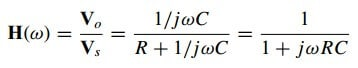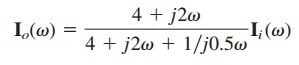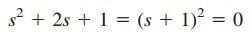# Learn What is Transfer Function QuicklyThe transfer function H(ω) (also called the network function) is a useful analytical tool for finding the frequency response of a circuit. You will find this function quite often when you try to analyze a closed-loop electrical circuit.

Contents

In fact, the frequency response of a circuit is the plot of the circuit’s transfer function H(ω) versus ω, with ω varying from ω = 0 to ω = ∞.

## What is Transfer Function

A transfer function is the frequency-dependent ratio of a forced function to a forcing function (or of output to input). The idea of a transfer function was implicit when we used the concepts of impedance and admittance to relate voltage and current. In general, a linear network can be represented by the block diagram shown in Figure.(1).

The transfer function H( ω) of a circuit is the frequency-dependent ratio of a phasor output Y( ω) (an element voltage or current) to a phasor input X( ω) (source voltage or current).

Thus,

assuming zero initial conditions. Since the input and output can be either voltage or current at any place in the circuit, there are four possible transfer functions:

where subscripts i and o denote input and output values. Being a complex quantity, H(ω) has a magnitude H (ω) and a phase φ; that is, H(ω) = H(ω)∠φ.

To obtain the transfer function using Equation.(2), we first obtain the frequency-domain equivalent of the circuit by replacing resistors, inductors, and capacitors with their impedances R, jωL, and 1/jωC.

We then use any circuit technique(s) to obtain the appropriate quantity in Equation.(2). We can obtain the frequency response of the circuit by plotting the magnitude and phase of the transfer function as the frequency varies.

A computer is a real time-saver for plotting the transfer function.

Some authors use H( jω) for transfer instead of H(ω), since ω and j are an inseparable pair.

The transfer function H(ω) can be expressed in terms of its numerator polynomial N(ω) and denominator polynomial D(ω) as

where N(ω) and D(ω) are not necessarily the same expressions for the input and output functions, respectively.

The representation of H(ω) in Equation.(3) assumes that common numerator and denominator factors in H(ω) have cancelled, reducing the ratio to lowest terms.

The roots of N(ω) = 0 are called the zeros of H(ω) and are usually represented as = z1, z2, . . . .

Similarly, the roots of D(ω) = 0 are the poles of H(ω) and are represented as = p1, p2, . . . .

A zero, as a root of the numerator polynomial, is a value that results in a zero value of the function. A pole, as a root of the denominator polynomial, is a value for which the function is infinite.

To avoid complex algebra, it is expedient to replace temporarily with s when working with H(ω) and replace s with at the end.

A zero may also be regarded as the value of s = jω that makes H(s) zero, and a pole as the value of s = jω that makes H(s) infinite.

## Transfer Function Examples

Let’s review the transfer function examples below:

### Transfer Function Example 1

For the RC circuit in Figure.(2a), obtain the transfer function Vo/Vs and its frequency response. Let vs = Vm cos ωt.

Solution:
The frequency-domain equivalent of the circuit is in Figure.(2b). By voltage division, the transfer function is given byWe obtain the magnitude and phase of H(ω) aswhere ω0 = 1/RC. To plot H and φ for 0 < ω < ∞, we obtain their values at some critical points and then sketch.

At ω = 0, H = 1 and φ = 0. At ω = ∞, H = 0 and φ = −90◦. Also, at ω = ω0, H = 1/√2 and φ = −45◦. With these and a few more points as shown in Table.(1), we find that the frequency response is as shown in Figure.(3).

### Transfer Function Example 2

For the circuit in Fig. 14.6, calculate the gain Io(ω)/Ii (ω) and its poles
and zeros.

Solution:
By current division,orThe zeros are atThe poles are atThus, there is a repeated pole (or double pole) at p = −1.